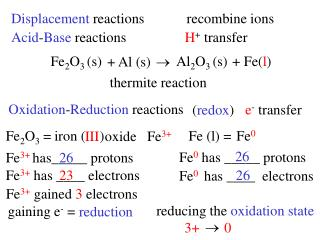# Acid - Base reactions - PowerPoint PPT PresentationDownload PresentationAcid - Base reactions

Presentation Description
Download Presentation## Acid - Base reactions

- - - - - - - - - - - - - - - - - - - - - - - - - - - E N D - - - - - - - - - - - - - - - - - - - - - - - - - - -
##### Presentation Transcript

1. Displacement reactions recombine ions Acid-Base reactions H+ transfer Fe2O3 (s) Al2O3 (s) + Fe (l) + Al (s)  thermite reaction Oxidation-Reduction reactions e- transfer (redox) Fe2O3 = iron ( ) Fe (l) = Fe0 III oxide Fe3+ 26 Fe0 has _____ protons Fe3+ has_____ protons 26 Fe3+ has ____ electrons 23 26 Fe0 has ____ electrons Fe3+ gained 3 electrons reducing the oxidation state gaining e- = reduction  3+ 0

2. Fe2O3 (s) Al2O3 (s) + Fe (l) + Al (s)  Al2O3 aluminum oxide Al (s) = Al0 Al0has _____ electrons 10 13 Al3+has ______ electrons 3+ oxidation state = 0 oxidation state = Al0 loses___ e- 3 losing e- = oxidation increase oxidation number  3+ 0 LEO GER Lose Electrons Oxidation Gain Electrons Reduction

3. Rules for oxidation numbers 1. Oxidation number for elements is zero. N2, O2, Na(s), Co(s), He (g) • Oxidation number of monatomic ions • is the same as their charge Group IA = +1 Al3+, Zn2+, Cd2+, Ag+ Group IIA = +2 • Oxidation number of oxygen in most compounds • is –2. Exceptions: H2O2, O2- (peroxides) –1 4. Oxidation number of hydrogen is +1 Exceptions: bonded to metals LiH 5. Fluorine is always –1. Other halogens are –1. Exceptions: bonded to O, they are positive

4. Assign oxidation numbers to all of the elements: Li2O Li = +1 O = -2 PF3 P = +3 F = -1 HNO3 H = +1 +5 O = -2 N = MnO4- Mn = +7 O = -2 Cr2O72- Cr = +6 -2 O =

5. What is the oxidation state of the highlighted element? P2O5 +5 diphosphorous pentoxide NaH -1 sodium hydride SnBr4 +4 tin (IV) bromide BaO2 -1 barium peroxide

6. Redox reactions 0 +1 0 +2  H2 (g) + 2H+ (aq) + Zn Zn (s) 2+  Zn oxidation state 0 +2 increase lose electrons oxidized reducing agent  H oxidation state +1 0 decrease gain electrons reduced oxidizing agent

7. 0 0 +1 -2 + O2 (g) 2H2O(l)  2H2(g) H loses or gains electrons is oxidized or reduced oxidizing or reducing agent O loses or gains electrons is oxidized or reduced oxidizing or reducing agent

8. 0 +7 +3 +4 + Al(s) + MnO4-  Al(OH)4- + MnO2 2H2O H2O What is being oxidized? Al What is oxidation? MnO4- Al(OH)4- LEO MnO2 How many e-? What is being reduced? GER What is the reducing agent?

9. What is the oxidation state of S in each of these compounds: H2S H = S = -2 +1 S8 S = 0 SCl2 S = +2 -1 Cl = Na2SO3 S = +4 O = -2 Na = +1 SO42- S = +6 -2 O = Which of these compounds can not act as a reducing agent? Which of these compounds can not act as a oxidizing agent?

10. +1 0 +4 -2 +1 -2 +2 -2 +2 -2 +1 Cd(OH)2 + Ni(OH)2 + NiO2 + 2H2O  Cd(s) What is being oxidized? What is being reduced? What is the oxidizing agent? What is the reducing agent? How many electrons are transferred?